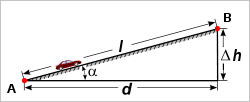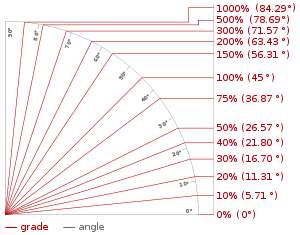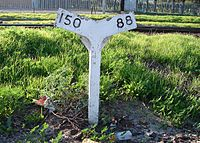d = run
Δh = rise
l = slope length
α = angle of inclination

The grade (also called slope, incline, gradient, pitch or rise) of a physical feature, topographic landform or constructed element, refers to the amount of inclination of that surface to the horizontal. It is a special case of the gradient in calculus where zero indicates gravitational level. A larger number indicates higher or steeper degree of "tilt". Often slope is calculated as a ratio of "rise" to "run", or as a fraction ("rise over run") in which run is the horizontal distance and rise is the vertical distance.

Grade or slope is applied to measuring existing physical features (such as canyon and hillsides, stream and river banks and beds), or in designing and engineering new elements for construction (such as roads, landscape and garden grading, roof pitches, railroads, aqueducts, and pedestrian-handicapped-bicycle circulation routes).

## Expression nomenclatureIllustration of grades in percent and angles in degrees

There are several systems for expressing slope:

1. as an angle of inclination to the horizontal. (This is the angle α opposite the "rise" side of a triangle with a right angle between vertical rise and horizontal run.)
2. as a percentage, the formula for which is$100 \frac{\text{rise}}{\text{run}}$ which could also be expressed as the tangent of the angle of inclination times 100. In the U.S., this percentage "grade" is the most commonly used unit for communicating slopes in transportation (streets, roads, highways and rail tracks), surveying, construction, and civil engineering.
3. as a per mille figure, the formula for which is$1000 \frac{\text{rise}}{\text{run}}$ which could also be expressed as the tangent of the angle of inclination times 1000. This is commonly used in Europe to denote the incline of a railway.
4. as a ratio of one part rise to so many parts run. For example, a slope that has a rise of 5 feet for every 100 feet of run would have a slope ratio of 1 in 20. (The word "in" is normally used rather than the mathematical ratio notation of "1:20"). This is generally the method used to describe railway grades in Australia and the UK.

Any one of these expressions may be used interchangeably to express the characteristics of a slope. Grade is usually expressed as a percentage, but this may easily be converted to the angle α from horizontal since that carries the same information.

There is a method in which slope may be expressed when the horizontal run is not known: rise divided by the hypotenuse (the slope length). This is not a usual way to measure slope. This follows the sine function rather than the tangent function and this method diverges from the "rise over run" method as angles start getting larger (see small-angle formula).

Many of the mathematical principles of slope that follow from the definition are applicable in topographic practice. In the UK, for road signs, maps and construction work, the gradient was traditionally expressed as a ratio such as 1 in 12, but signs showing gradient expressed as a percentage are becoming more common.

In civil engineering applications and physical geography, the slope is calculated along a particular direction of interest which is normally the route of a highway or railway road bed.

### Mathematical equations

Grades can be related using the following equations with symbols from the figure at top.

Tangent as a ratio$\tan{\alpha} = \frac{\Delta h}{d}$
This ratio can also be expressed as a percentage by multiplying by 100.$\alpha = \arctan{\frac{\Delta h}{d}}$
If the tangent is expressed as a percentage, the angle can be determined as:$\alpha = \arctan{\frac{\%\,\text{slope}}{100}}$

If the angle is expressed as a ratio (1 in n) then:$\alpha = \arctan{\frac{1}{n}}$

In vehicular engineering, various land-based designs (cars, SUVs, trucks, trains, etc.) are rated for their ability to ascend terrain. (Trains typically rate much lower than cars.) The highest grade a vehicle can ascend while maintaining a particular speed is sometimes termed that vehicle's "gradeability" (or, less often, "grade ability"). The lateral slopes of a highway geometry are sometimes called fills or cuts where these techniques have been used to create them.

## Environmental design

Grade, pitch, and slope are important components in landscape design, garden design, landscape architecture, and architecture; for engineering and aesthetic design factors. Drainage, slope stability, circulation of people and vehicles, complying with building codes, and design integration are aspects of slope considerations in environmental design.

## RailwaysGrade indicator near Bellville, Western Cape, South Africa, showing 1:150 and 1:88 grades.

Gradients limit the load that a locomotive can haul, including the weight of the locomotive itself. On a 1% gradient (1 in 100) a locomotive can pull half (or less) of the load that it can pull on level track. (A heavily loaded train rolling at 20 km/hr on heavy rail may require ten times the pull on a 1% upgrade that it does on the level at that speed.) Early railways in the United Kingdom were laid out with very gentle gradients, such as 0.05% (1 in 2000), because the early locomotives (and their brakes) were so feeble. Steep gradients were concentrated in short sections of lines where it was convenient to employ assistant engines or cable haulage, such as from Euston to Camden Town, about 1.2 km. Extremely steep gradients need the help of cables, or some kind of rack railway.

Gradients can be expressed as W feet per mile, X feet per chain, 1 in Y, and Z%. Since surveyors like round figures, the method of express can affect the gradients selected.

The steepest railway lines that do not utilize a rack system include:

It is customary for civil engineers to refer to the steepest grade on a section of rail line as the ruling grade for that section. Civil engineering works such as cuttings, embankments and tunnels are employed to reduce this maximum grade.

### Compensation for curvature

Gradients on sharp curves are effectively a bit steeper than the same gradient on straight track, so to "compensate" for this and make the ruling grade uniform throughout, the gradient on those sharp curves should be reduced slightly.

### Continuous brakes

In the era before continuous brakes, whether air brake or vacuum brakes steep gradients were a serious problem making it difficult to stop on the grade safely. In an extreme example, the Inspector insisted that a station be regraded before he would allow it to open. This required the gradient through the platform to be eased from 1 in 80 to 1 in 130.

The greater a grade, the more power an animal or a machine requires to climb it at a given speed; therefore routes with lower grades are preferred, so long as they do not have other disadvantages, such as causing the overall travel distance to increase significantly.

Vehicles proceeding up a steep grade demand more fuel consumption with typically increased air pollution generation. Sound level increases are also produced by motor vehicles travelling upgrade.

Wikimedia Foundation. 2010.

### Look at other dictionaries:

• Slope — is used to describe the steepness, incline, gradient, or grade of a straight line. A higher slope value indicates a steeper incline. The slope is defined as the ratio of the rise divided by the run between two points on a line, or in other words …   Wikipedia

• Grade — NOTOC Grade may refer to:In education* Grade (education), a teacher s evaluation of a student s performance * Grade level, the numbering of the year a student has reached in school.In civil engineering* Grade (slope), the pitch of a slope such as …   Wikipedia

• grade — [grād] n. [Fr < L gradus, a step, degree, rank < gradi, to step, walk < IE base * ghredh , to stride > Goth griths, step] 1. any of the stages in an orderly, systematic progression; step; degree 2. a) a degree or rating in a scale… …   English World dictionary

• grade — [n1] rank, step brand, caliber, category, class, classification, condition, degree, division, echelon, estate, form, gradation, group, grouping, league, level, mark, notch, order, pigeonhole*, place, position, quality, rung*, size, stage,… …   New thesaurus

• grade — grade1 W3S3 [greıd] n ▬▬▬▬▬▬▬ 1¦(standard)¦ 2¦(rank)¦ 3¦(mark in school)¦ 4 make the grade 5¦(school year)¦ 6¦(slope)¦ ▬▬▬▬▬▬▬ [Date: 1500 1600; : French; Origin: Latin gradus step, d …   Dictionary of contemporary English

• grade — /grayd/, n., v., graded, grading. n. 1. a degree or step in a scale, as of rank, advancement, quality, value, or intensity: the best grade of paper. 2. a class of persons or things of the same relative rank, quality, etc. 3. a step or stage in a… …   Universalium

• grade —    1. a unit of angle measurement equal to 1/400 circle, 0.01 right angle, 0.9°, or 54 . This unit was introduced in France, where it is called the grade, in the early years of the metric system. The grad is the English version, apparently… …   Dictionary of units of measurement

• grade — I (New American Roget s College Thesaurus) n. level, quality, class; rank, standing; gradation, slope, tilt, slant. See obliquity, degree. II (Roget s IV) n. 1. [An incline] Syn. hill, slope, inclined plane, gradient, slant, inclination, pitch,… …   English dictionary for students

• grade — [[t]greɪd[/t]] n. v. grad•ed, grad•ing 1) a degree or step in a scale, as of rank, advancement, quality, value, or intensity 2) a class of persons or things of the same relative rank, quality, etc 3) a step or stage in a course or process 4) edu… …   From formal English to slang

• grade — 1 /greId/ noun 1 STANDARD (C, U) a particular standard or level of quality that a product, material etc has: Grade A eggs | low grade steel 2 COMPANY (C) the level of importance you have or the level of pay you receive in a company or… …   Longman dictionary of contemporary English